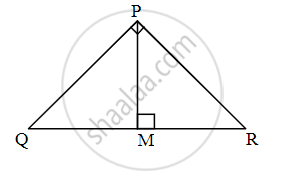# From given figure, in ∆PQR, if ∠QPR = 90°, PM ⊥ QR, PM = 10, QM = 8, then for finding the value of QR, complete the following activity.Activity: In ∆PQR, if ∠QPR = 90°, PM ⊥ QR, ......[Given] In ∆PM - Geometry

Sum

From given figure, in ∆PQR, if ∠QPR = 90°, PM ⊥ QR, PM = 10, QM = 8, then for finding the value of QR, complete the following activity.Activity: In ∆PQR, if ∠QPR = 90°, PM ⊥ QR,  ......[Given]

In ∆PMQ, by Pythagoras Theorem,

∴ PM2 + square = PQ2     ......(I)

∴ PQ2 = 102 + 82

∴ PQ2 = square + 64

∴ PQ2 = square

∴ PQ = sqrt(164)

Here, ∆QPR ~ ∆QMP ~ ∆PMR

∴ ∆QMP ~ ∆PMR

∴ "PM"/"RM" = "QM"/"PM"

∴ PM2 = RM × QM

∴ 102 = RM × 8

RM = 100/8 = square

And,

QR = QM + MR

QR = square + 25/2 = 41/2

#### Solution

In ∆PQR, if ∠QPR = 90°, PM ⊥ QR,  ......[Given]

In ∆PMQ,

by Pythagoras Theorem,

∴ PM2 + QM2 = PQ2     ......(I)

∴ PQ2 = 102 + 82

∴ PQ2 = 100 + 64

∴ PQ2 = 164

∴ PQ = sqrt(164)

Here, ∆QPR ~ ∆QMP ~ ∆PMR

∴ ∆QMP ~ ∆PMR

∴ "PM"/"RM" = "QM"/"PM"   ......[Corresponding sides of similar triangles]

∴ PM2 = RM × QM

∴ 102 = RM × 8

RM = 100/8 = 25/2

And,

QR = QM + MR

QR = 8 + 25/2 = 41/2

Concept: Similarity in Right Angled Triangles
Is there an error in this question or solution?

Share